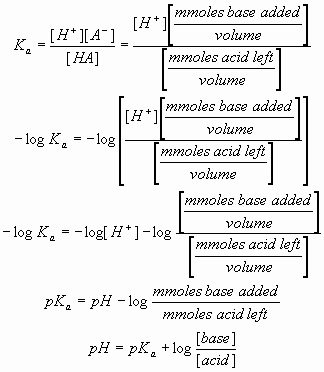# Multiple Choice Questions on pH and Buffer

26. The Henderson-Hasselbalch equation:a) allows the graphic determination of the molecular weight of a weak acid from its pH alone.
b) does not explain the behavior of di- or tri-basic weak acids
c) employs the same value for pKa for all weak acids.
d) is equally useful with solutions of acetic acid and of hydrochloric acid.
e) relates the pH of a solution to the pKa and the concentrations of acid and conjugate base.

27. Consider an acetate buffer, initially at the same pH as its pKa (4.76). When sodium hydroxide (NaOH) is mixed with this buffer, the:
a) pH remains constant.
b) pH rises more than if an equal amount of NaOH is added to an acetate buffer initially at pH 6.76.
c) pH rises more than if an equal amount of NaOH is added to unbuffered water at pH 4.76.
d) ratio of acetic acid to sodium acetate in the buffer falls.
e) sodium acetate formed precipitates because it is less soluble than acetic acid.

28. A compound is known to have a free amino group with a pKa of 8.8, and one other ionizable group with a pKa between 5 and 7. To 100 mL of a 0.2 M solution of this compound at pH 8.2 was added 40 mL of a solution of 0.2 M hydrochloric acid. The pH changed to 6.2. The pKa of the second ionizable group is:
a) The pH cannot be determined from this information.
b) 5.4
c) 5.6
d) 6.0
e) 6.2

29. Three buffers are made by combining a 1 M solution of acetic acid with a 1 M solution of sodium acetate in the ratios shown below.
###### 1 M acetic acid   1 M sodium acetate
Buffer 1:       10 mL           90 mL
Buffer 2:       50 mL           50 mL
Buffer 3:       90 mL          10 mL
Which of these statements is true of the resulting buffers?
a) pH of buffer 1 < pH of buffer 2 < pH of buffer 3
b) pH of buffer 1 = pH of buffer 2 = pH of buffer 3
c) pH of buffer 1 > pH of buffer 2 > pH of buffer 3
d) The problem cannot be solved without knowing the value of pKa.
e) None of the above.

30. A 1.0 M solution of a compound with 2 ionizable groups (pKa’s = 6.2 and 9.5; 100 mL total) has a pH of 6.8. If a biochemist adds 60 mL of 1.0 M HCl to this solution, the solution will change to pH:
a) 5.60
b) 8.90
c) 9.13
d) 9.32
e) The pH cannot be determined from this information.

Answers:
26. e) relates the pH of a solution to the pKa and the concentrations of acid and conjugate base.
27. d) ratio of acetic acid to sodium acetate in the buffer falls.
28. c) 5.6
29. b) pH of buffer 1 = pH of buffer 2 = pH of buffer 3
30. a) 5.60

Reference: Lehninger Principles of Biochemistry Lecture Notebook 4th Revised Edition

### 2 Comments

We love to hear from you! Leave us a comment.

1.The answer to 29 is incorrect. Should be C.

2.question 29, answer would be option c.
5.69>4.74>3.78
pH OF BUFFER 1>pH OF BUFFER 2>pH OF BUFFER 3.